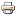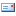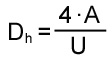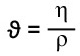# What is the Reynolds number 2The Reynolds number is named after the physicist Osborne Reynolds. The Reynolds number is used in fluid mechanics to determine the type of flow. For a better understanding, the different types of flow are described first.

### Types of flow

In fluid mechanics, there are two different types of flow that can occur with fluids (liquids or gases): the laminar flow and the turbulent flow.

Laminar flow:
In the laminar flow of liquids and gases, there is no turbulence (i.e. eddies / cross flows). This means that the fluid flows in layers that do not mix.

Turbulent flow:
In turbulent flow, in contrast to laminar flow, eddies occur.In hydraulics, assuming that the fluid flows in a smooth round pipe, the following values ​​for the Reynolds number can be assumed:

Recrit = 2200 to 2300
Re> Recrit => The flow is turbulent
Re crit => Flow is laminar

### Calculate Reynolds number

The Reynolds number is calculated from the ratio of inertia to viscosity forces. The calculation includes the mean flow velocity [m / s], the hydraulic diameter [m] and the kinematic viscosity [m2/be. The Reynolds number itself has no unit.The Reynolds number results from the following formula:

vm : mean flow velocity [m / s]
D.H : Hydraulic diameter [m]
ϑ: kinematic viscosity (toughness) [m2/ s]

> Viscosity

Calculation of the hydraulic diameterA: flow cross section
U: circumference of the flow cross-section

Calculation of the kinematic viscosityη: dynamic viscosity [kg / (s * m)]
ρ: density [kg / m3]

The kinematic viscosity in hydraulics is typically in the range between 10 and 100 mm2/ s

The Reynolds number will be of importance several times in the further course of this hydraulics script. It is necessary, for example, when calculating the pipe friction. Pipe friction is discussed in one of the following scripts.